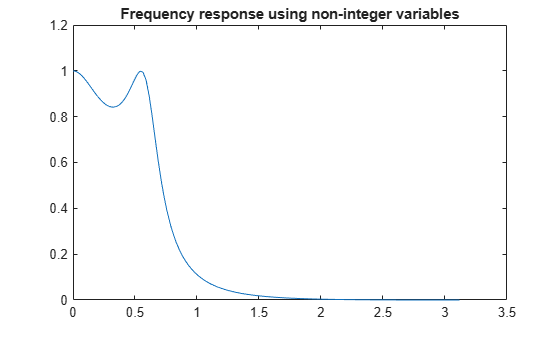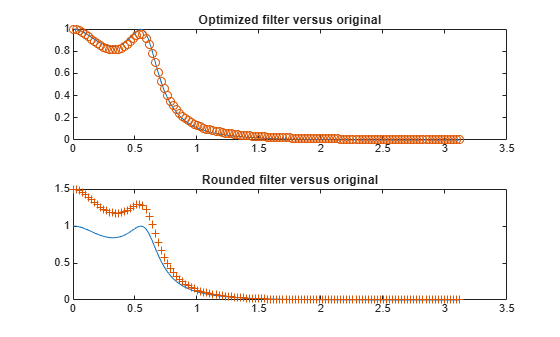# Minimax Optimization

This example shows how to solve a nonlinear filter design problem using a minimax optimization algorithm, `fminimax`, in Optimization Toolbox™. Note that to run this example you must have the Signal Processing Toolbox™ installed.

### Set Finite Precision Parameters

Consider an example for the design of finite precision filters. For this, you need to specify not only the filter design parameters such as the cut-off frequency and number of coefficients, but also how many bits are available since the design is in finite precision.

```nbits = 8; % How many bits have we to realize filter maxbin = 2^nbits-1; % Maximum number expressable in nbits bits n = 4; % Number of coefficients (order of filter plus 1) Wn = 0.2; % Cutoff frequency for filter Rp = 1.5; % Decibels of ripple in the passband w = 128; % Number of frequency points to take```

### Continuous Design First

This is a continuous filter design; we use `cheby1`, but we could also use `ellip`, `yulewalk` or `remez` here:

```[b1,a1] = cheby1(n-1,Rp,Wn); [h,w] = freqz(b1,a1,w); % Frequency response h = abs(h); % Magnitude response plot(w, h) title('Frequency response using non-integer variables')````x = [b1,a1]; % The design variables`

### Set Bounds for Filter Coefficients

We now set bounds on the maximum and minimum values:

```if (any(x < 0)) % If there are negative coefficients - must save room to use a sign bit % and therefore reduce maxbin maxbin = floor(maxbin/2); vlb = -maxbin * ones(1, 2*n)-1; vub = maxbin * ones(1, 2*n); else % otherwise, all positive vlb = zeros(1,2*n); vub = maxbin * ones(1, 2*n); end```

### Scale Coefficients

Set the biggest value equal to maxbin and scale other filter coefficients appropriately.

```[m, mix] = max(abs(x)); factor = maxbin/m; x = factor * x; % Rescale other filter coefficients xorig = x; xmask = 1:2*n; % Remove the biggest value and the element that controls D.C. Gain % from the list of values that can be changed. xmask(mix) = []; nx = 2*n;```

### Set Optimization Criteria

Using `optimoptions`, adjust the termination criteria to reasonably high values to promote short running times. Also turn on the display of results at each iteration:

```options = optimoptions('fminimax', ... 'StepTolerance', 0.1, ... 'OptimalityTolerance', 1e-4,... 'ConstraintTolerance', 1e-6, ... 'Display', 'iter');```

### Minimize the Absolute Maximum Values

We need to minimize absolute maximum values, so we set options.MinAbsMax to the number of frequency points:

```if length(w) == 1 options = optimoptions(options,'AbsoluteMaxObjectiveCount',w); else options = optimoptions(options,'AbsoluteMaxObjectiveCount',length(w)); end```

### Eliminate First Value for Optimization

Discretize and eliminate first value and perform optimization by calling FMINIMAX:

`[x, xmask] = elimone(x, xmask, h, w, n, maxbin)`
```x = 1×8 0.5441 1.6323 1.6323 0.5441 57.1653 -127.0000 108.0000 -33.8267 ```
```xmask = 1×6 1 2 3 4 5 8 ```
```niters = length(xmask); disp(sprintf('Performing %g stages of optimization.\n\n', niters));```
```Performing 6 stages of optimization. ```
```for m = 1:niters fun = @(xfree)filtobj(xfree,x,xmask,n,h,maxbin); % objective confun = @(xfree)filtcon(xfree,x,xmask,n,h,maxbin); % nonlinear constraint disp(sprintf('Stage: %g \n', m)); x(xmask) = fminimax(fun,x(xmask),[],[],[],[],vlb(xmask),vub(xmask),... confun,options); [x, xmask] = elimone(x, xmask, h, w, n, maxbin); end```
```Stage: 1 ```
``` Objective Max Line search Directional Iter F-count value constraint steplength derivative Procedure 0 8 0 0.00329174 1 17 0.0001845 3.34e-07 1 0.0143 Local minimum possible. Constraints satisfied. fminimax stopped because the size of the current search direction is less than twice the value of the step size tolerance and constraints are satisfied to within the value of the constraint tolerance. ```
```Stage: 2 ```
``` Objective Max Line search Directional Iter F-count value constraint steplength derivative Procedure 0 7 0 0.0414182 1 15 0.01649 0.0002558 1 0.261 2 23 0.01544 6.126e-07 1 -0.0282 Hessian modified Local minimum possible. Constraints satisfied. fminimax stopped because the size of the current search direction is less than twice the value of the step size tolerance and constraints are satisfied to within the value of the constraint tolerance. ```
```Stage: 3 ```
``` Objective Max Line search Directional Iter F-count value constraint steplength derivative Procedure 0 6 0 0.0716961 1 13 0.05943 2.6e-10 1 0.776 Local minimum possible. Constraints satisfied. fminimax stopped because the size of the current search direction is less than twice the value of the step size tolerance and constraints are satisfied to within the value of the constraint tolerance. ```
```Stage: 4 ```
``` Objective Max Line search Directional Iter F-count value constraint steplength derivative Procedure 0 5 0 0.129938 1 11 0.04278 -8.933e-10 1 0.183 Local minimum possible. Constraints satisfied. fminimax stopped because the size of the current search direction is less than twice the value of the step size tolerance and constraints are satisfied to within the value of the constraint tolerance. ```
```Stage: 5 ```
``` Objective Max Line search Directional Iter F-count value constraint steplength derivative Procedure 0 4 0 0.0901749 1 9 0.03867 -2.263e-10 1 0.256 Local minimum possible. Constraints satisfied. fminimax stopped because the size of the current search direction is less than twice the value of the step size tolerance and constraints are satisfied to within the value of the constraint tolerance. ```
```Stage: 6 ```
``` Objective Max Line search Directional Iter F-count value constraint steplength derivative Procedure 0 3 0 0.11283 1 7 0.05033 9.714e-17 1 0.197 Local minimum possible. Constraints satisfied. fminimax stopped because the size of the current search direction is less than twice the value of the step size tolerance and constraints are satisfied to within the value of the constraint tolerance. ```

### Check Nearest Integer Values

See if nearby values produce a better filter.

```xold = x; xmask = 1:2*n; xmask([n+1, mix]) = []; x = x + 0.5; for i = xmask [x, xmask] = elimone(x, xmask, h, w, n, maxbin); end xmask = 1:2*n; xmask([n+1, mix]) = []; x = x - 0.5; for i = xmask [x, xmask] = elimone(x, xmask, h, w, n, maxbin); end if any(abs(x) > maxbin) x = xold; end```

### Frequency Response Comparisons

We first plot the frequency response of the filter and we compare it to a filter where the coefficients are just rounded up or down:

```subplot(211) bo = x(1:n); ao = x(n+1:2*n); h2 = abs(freqz(bo,ao,128)); plot(w,h,w,h2,'o') title('Optimized filter versus original') xround = round(xorig)```
```xround = 1×8 1 2 2 1 57 -127 108 -34 ```
```b = xround(1:n); a = xround(n+1:2*n); h3 = abs(freqz(b,a,128)); subplot(212) plot(w,h,w,h3,'+') title('Rounded filter versus original')``````fig = gcf; fig.NextPlot = 'replace';```